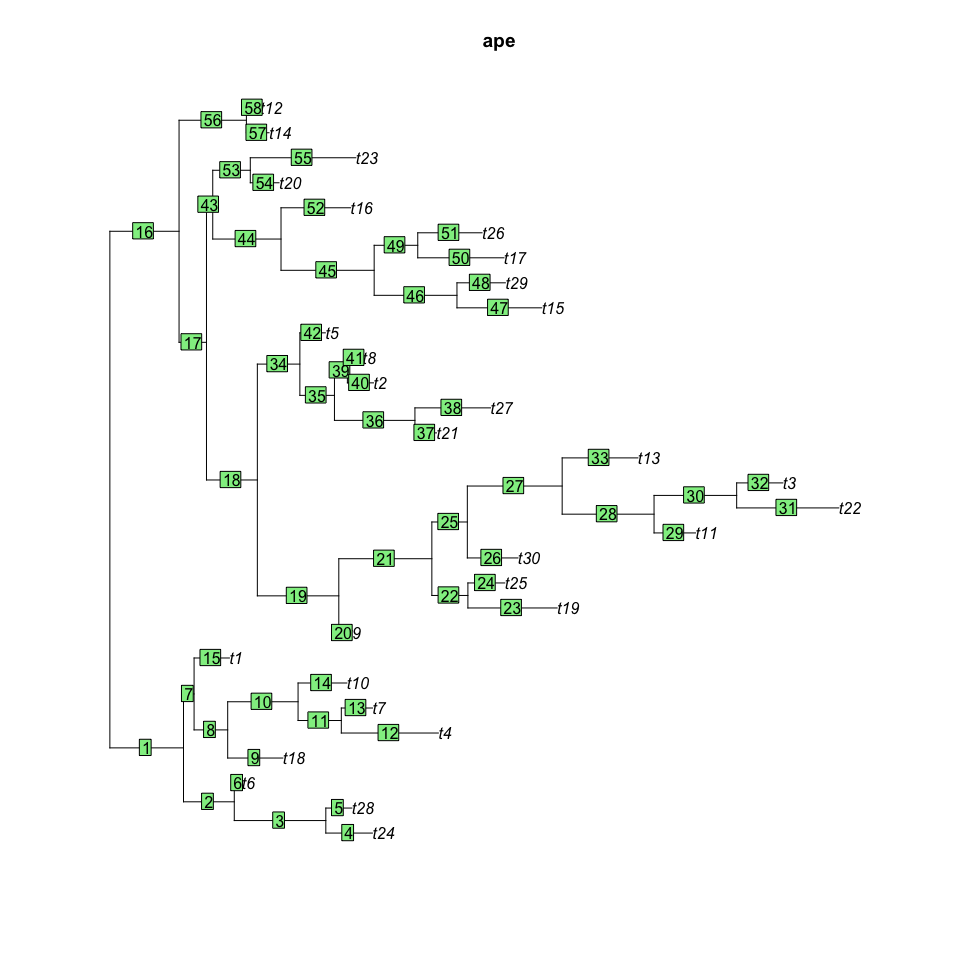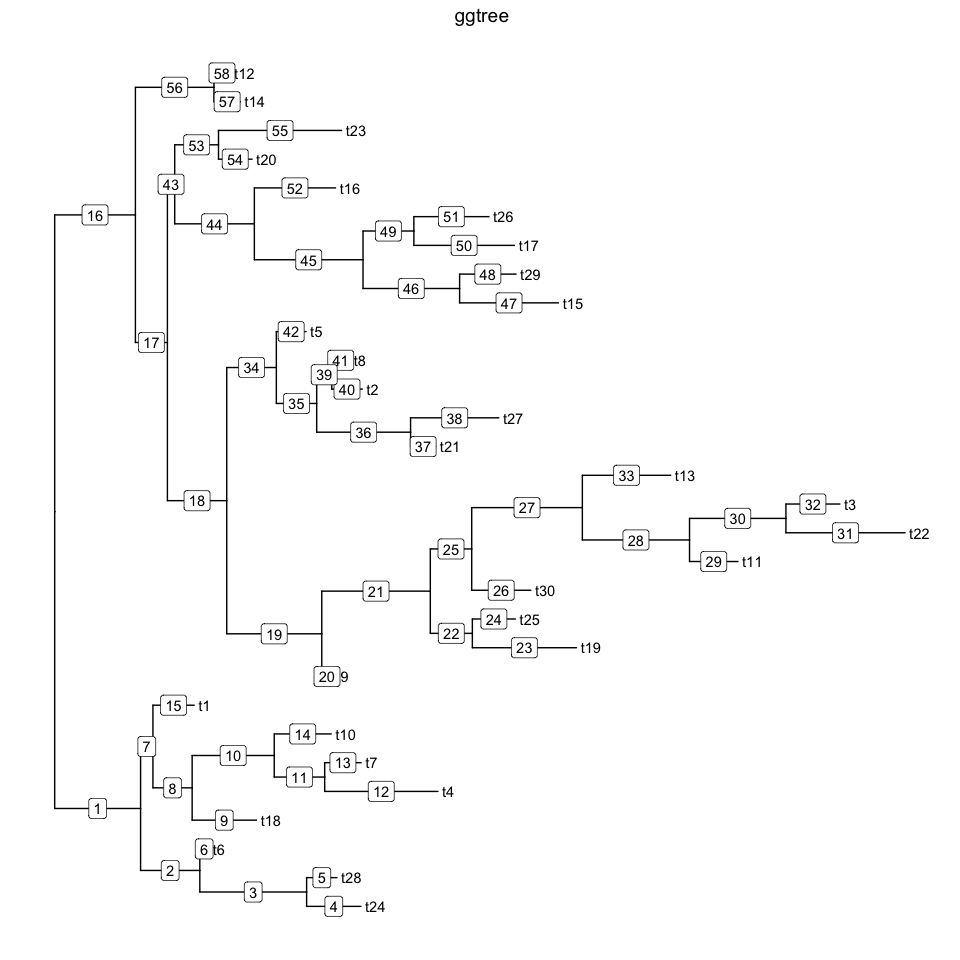This is a question from `ggtree` user. In `ape` and `phytools`, it’s easy to label edge using the `edgelabels` function.

``````set.seed(1)
tr = rtree(30)
library(ape)
plot(tr, main="ape")
edgelabels()
``````I don’t see any necessity to label edge numbers, as they are meaningless. The number is labeled as the row index of `tr\$edge`, and edge can be uniquely mapped to child node. If we need to relate something to edge, we can relate them to corresponding child node. Node is a central hub in tree annotation in `ggtree`.

To label edge number, we can also attach the edge number to node and then label them as a node attribute.

``````library(ggtree)
p = ggtree(tr, ladderize=F) + geom_tiplab() + ggtitle("ggtree")
edge=data.frame(tr\$edge, edge_num=1:nrow(tr\$edge))
colnames(edge)=c("parent", "node", "edge_num")
p %<+% edge + geom_label(aes(x=branch, label=edge_num))
``````## Citation

G Yu, DK Smith, H Zhu, Y Guan, TTY Lam*. ggtree: an R package for visualization and annotation of phylogenetic trees with their covariates and other associated data. Methods in Ecology and Evolution. `doi:10.1111/2041-210X.12628`.